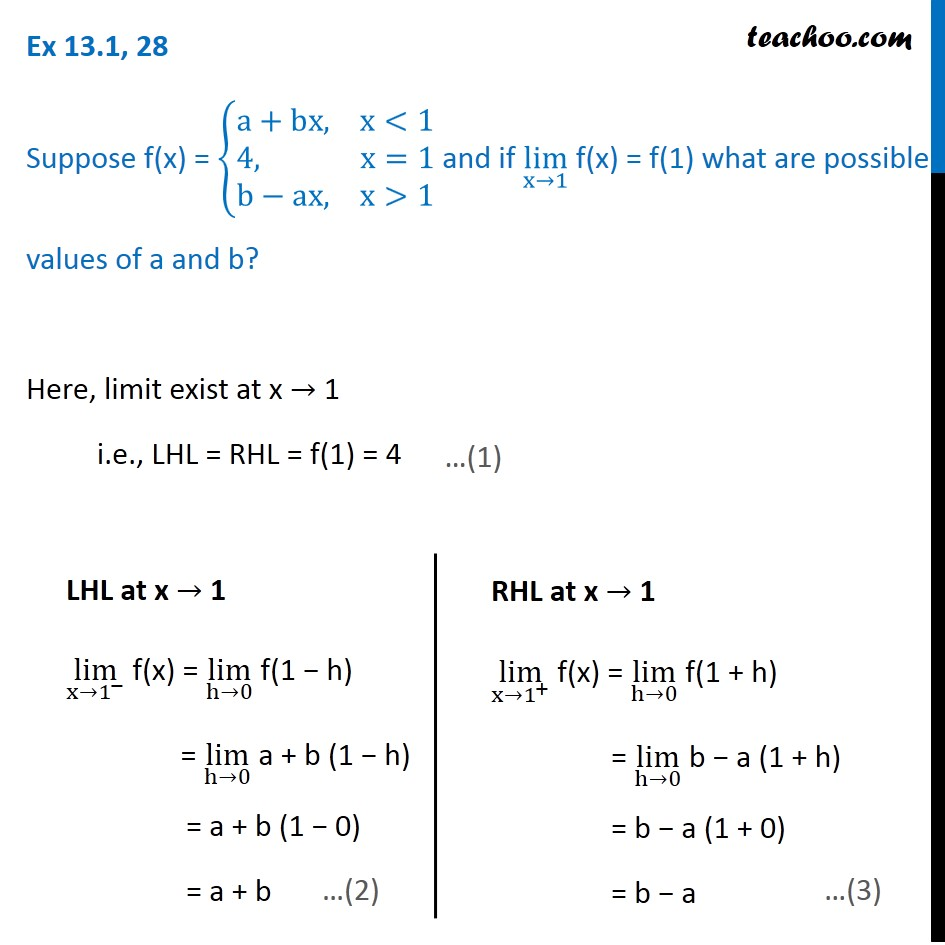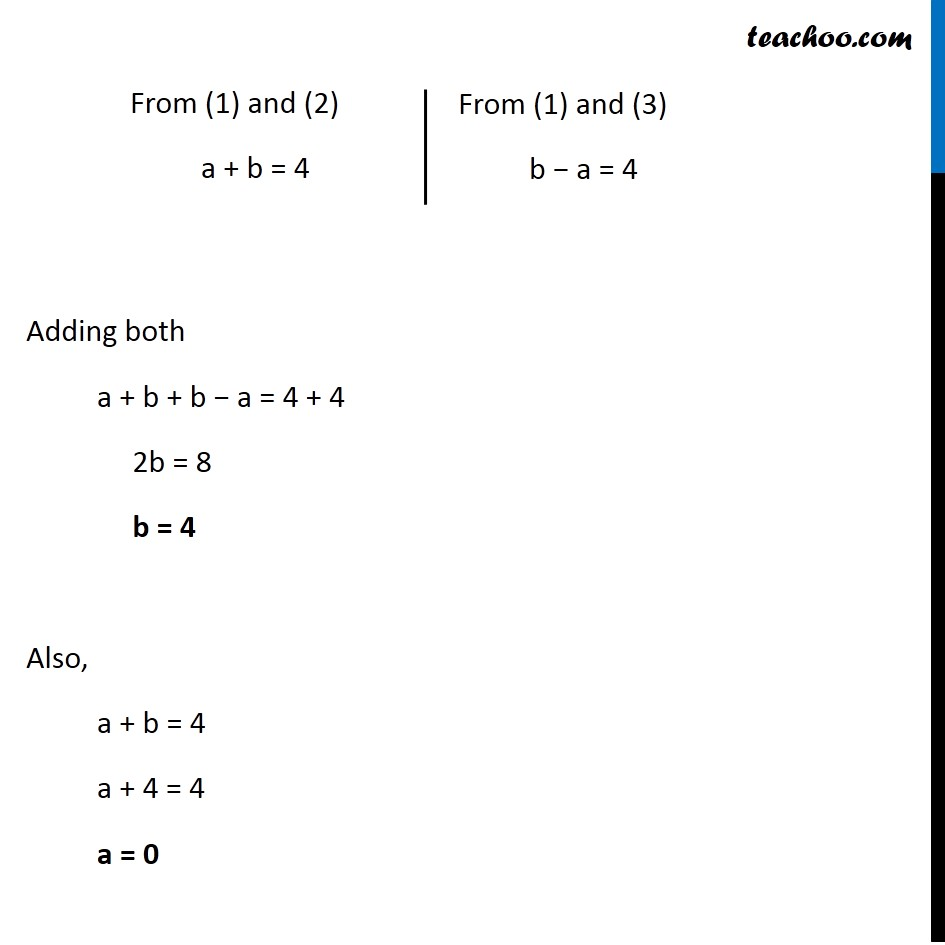1. Chapter 13 Class 11 Limits and Derivatives (Term 1 and Term 2)
2. Concept wise
3. Limits - Limit exists

Transcript

Ex 13.1, 28 Suppose f(x) = {█(a+bx, x<1@4, x=1@b−ax, x>1)┤ and if lim┬(x→1) f(x) = f(1) what are possible values of a and b? Here, limit exist at x → 1 i.e., LHL = RHL = f(1) = 4 LHL at x → 1 lim┬(x→1^− ) f(x) = lim┬(h→0) f(1 − h) = lim┬(h→0) a + b (1 − h) = a + b (1 − 0) = a + b RHL at x → 1 lim┬(x→1^+ ) f(x) = lim┬(h→0) f(1 + h) = lim┬(h→0) b − a (1 + h) = b − a (1 + 0) = b − a From (1) and (2) a + b = 4 From (1) and (3) b − a = 4 Adding both a + b + b − a = 4 + 4 2b = 8 b = 4 Also, a + b = 4 a + 4 = 4 a = 0